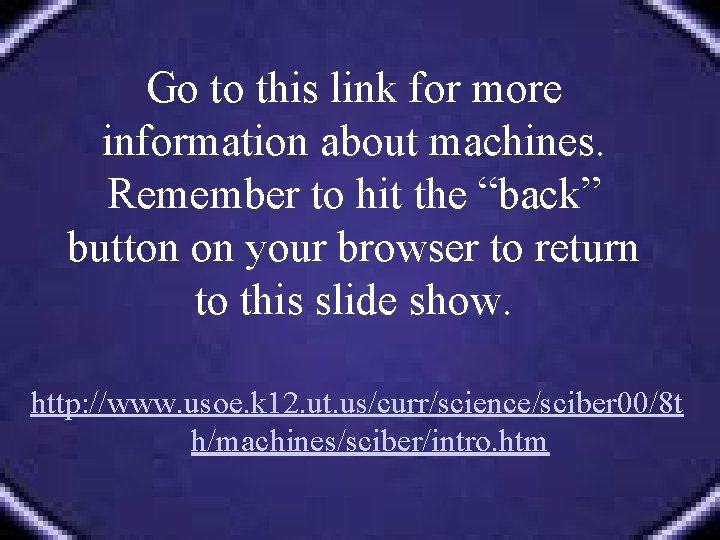# Work Simple Machines Review Define Describe WORK Define

• Slides: 47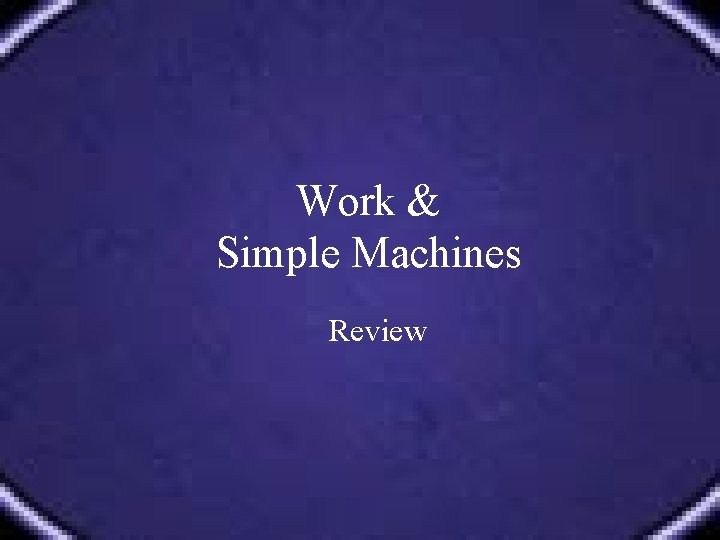Work & Simple Machines Review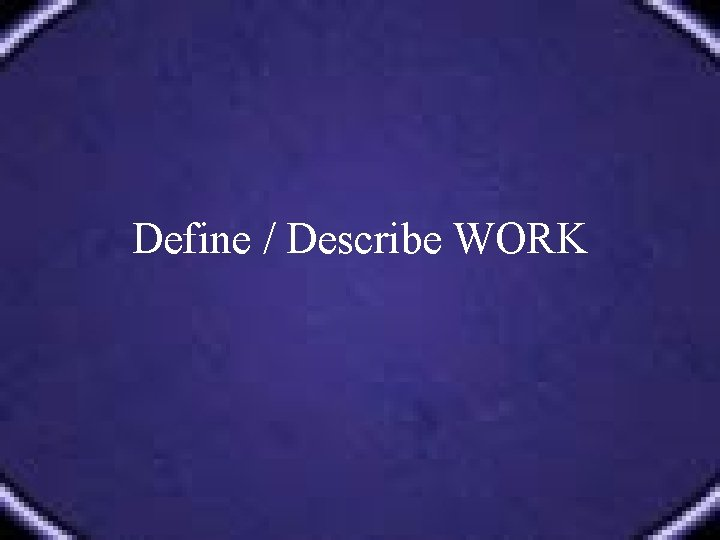Define / Describe WORKDefine / Describe WORK • Work is done when a force causes an object to move in the direction that the force is applied. • The formula for work is: W = F X D • If there is no movement, there is no work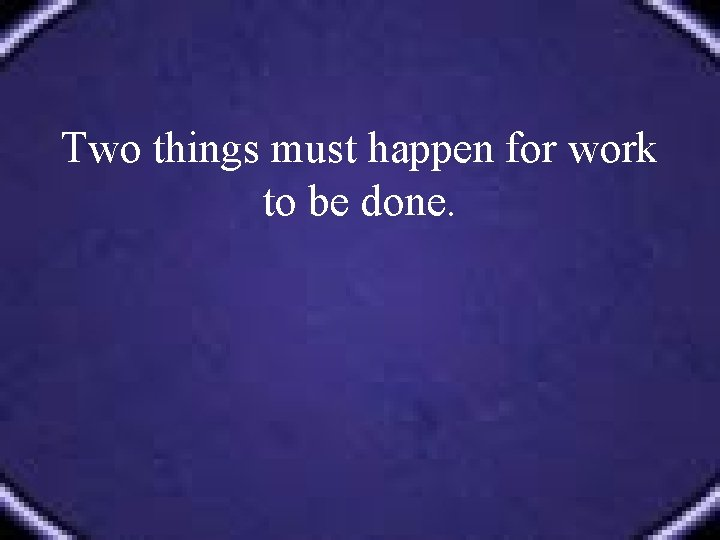Two things must happen for work to be done.Two things must happen for work to be done. • A force must applied to an object • The object must move in the same direction as the force.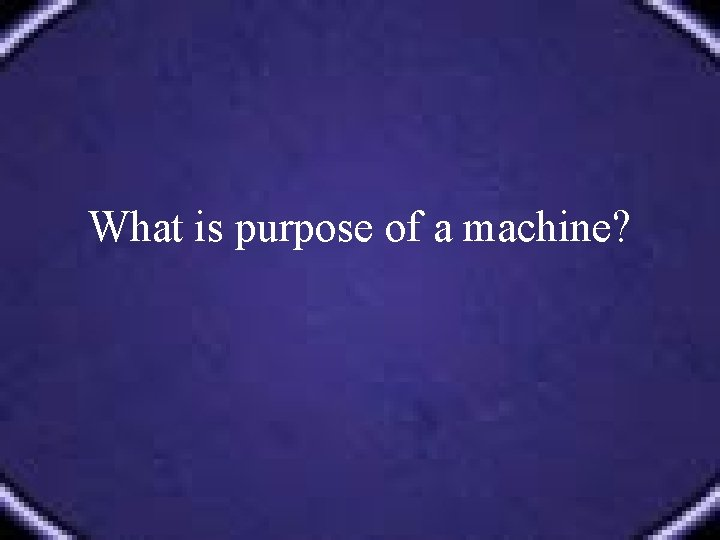What is purpose of a machine?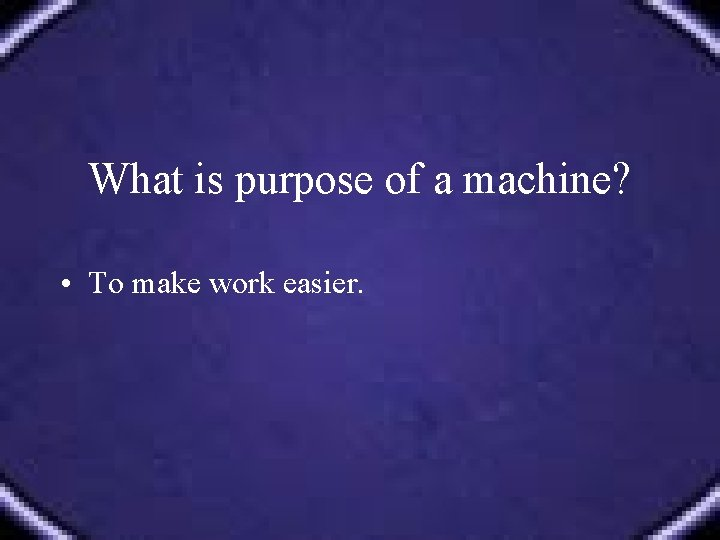What is purpose of a machine? • To make work easier.How do machines make work easier?How do machines make work easier? • They can change the amount of force • They can change the distance (Remember that Work = Force X Distance) • They can also change the direction MOREHow do machines make work easier? Any change in the size of the force changes the distance. No machine can increase both force and distance. 1. Multiply force which decreases distance the load moves. 2. Multiply the distance which decreases force. 3. Or, they leave force and distance alone but change the direction in which the load moves.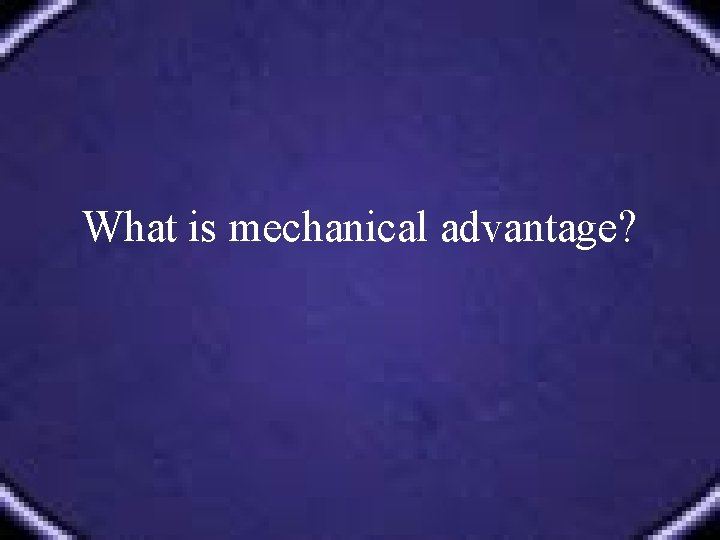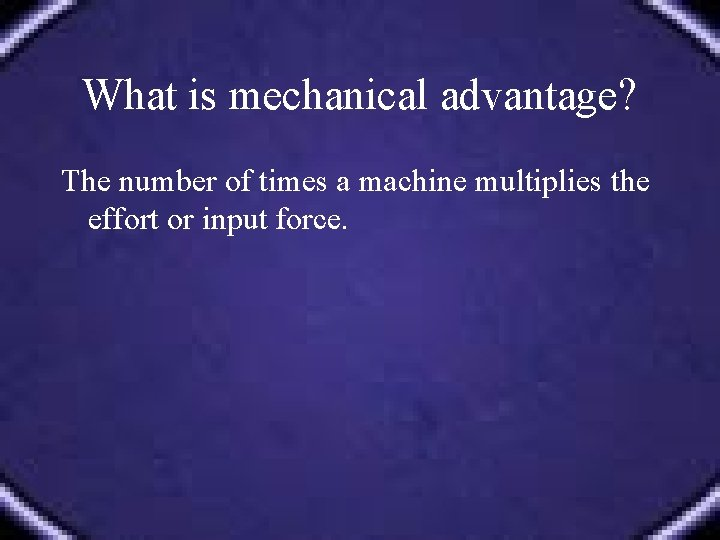What is mechanical advantage? The number of times a machine multiplies the effort or input force.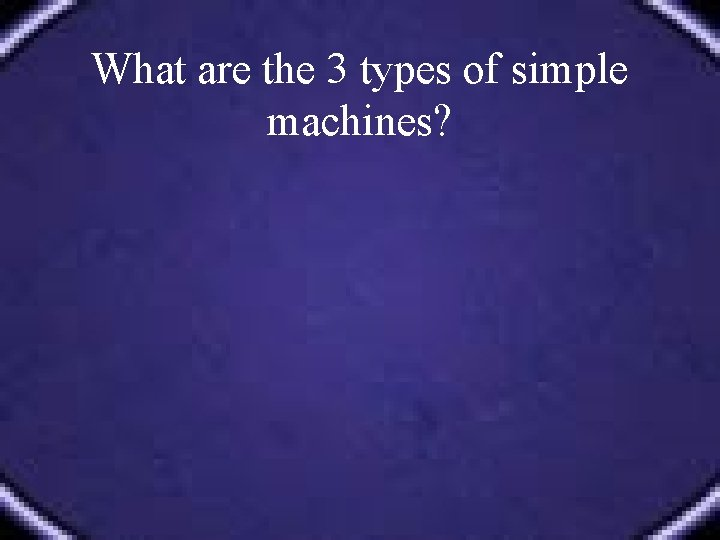What are the 3 types of simple machines?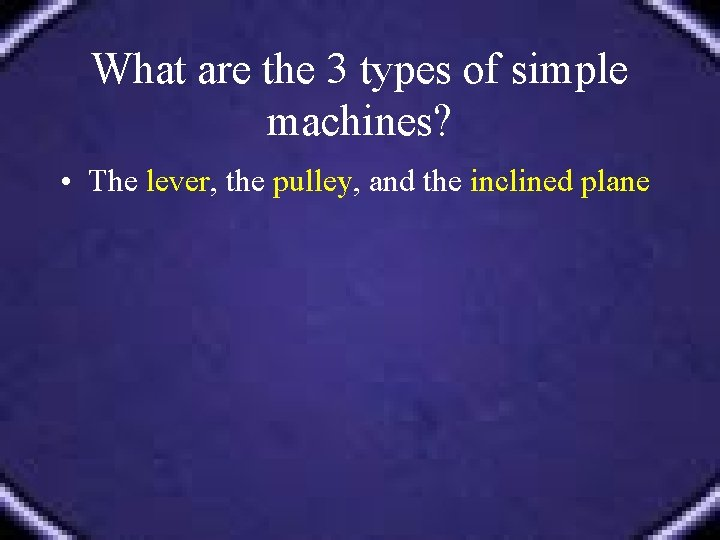What are the 3 types of simple machines? • The lever, the pulley, and the inclined plane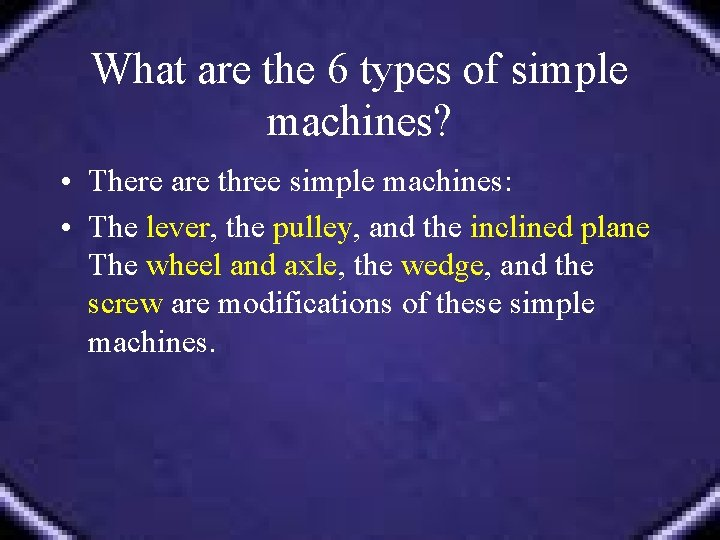What are the 6 types of simple machines? • There are three simple machines: • The lever, the pulley, and the inclined plane The wheel and axle, the wedge, and the screw are modifications of these simple machines.What is a compound (or complex) machine?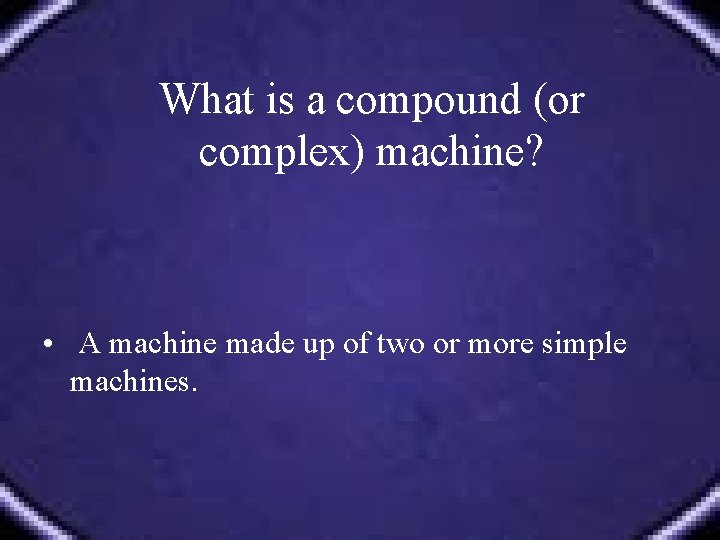What is a compound (or complex) machine? • A machine made up of two or more simple machines.Describe LEVERDescribe LEVER A simple machine made with a rigid bar free to pivot (move) around a fixed point called a fulcrum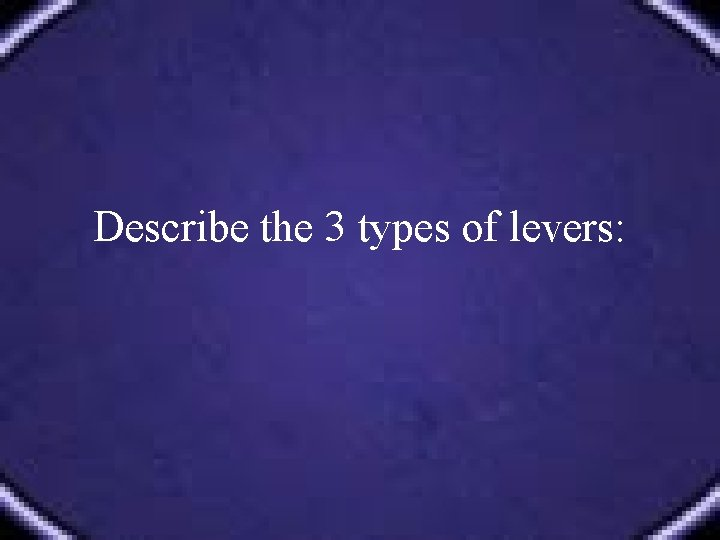Describe the 3 types of levers:Describe the 3 types of levers: • A first class lever is like a teeter-totter or see -saw. One end will lift an object (child) up just as far as the other end is pushed down. • A second class lever is like a wheel barrow. The long handles of a wheel barrow are really the long arms of a lever. • A third class lever is like a fishing pole. When the pole is given a tug, one end stays still but the other end flips in the air catching the fish. MORE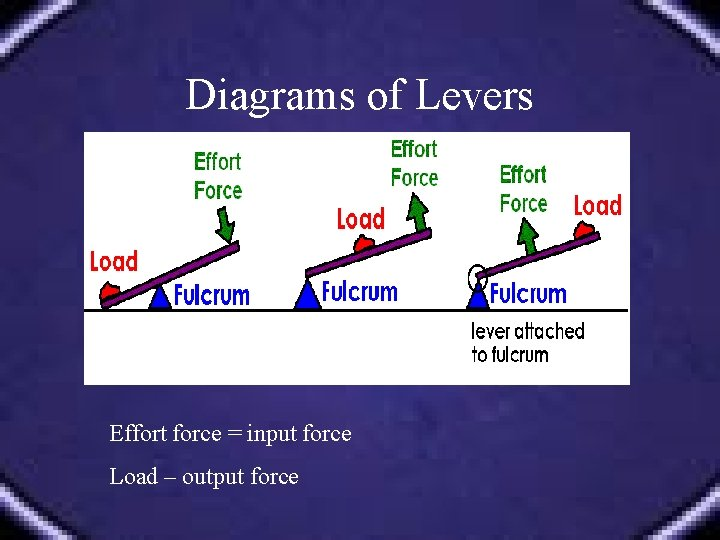Diagrams of Levers Effort force = input force Load – output force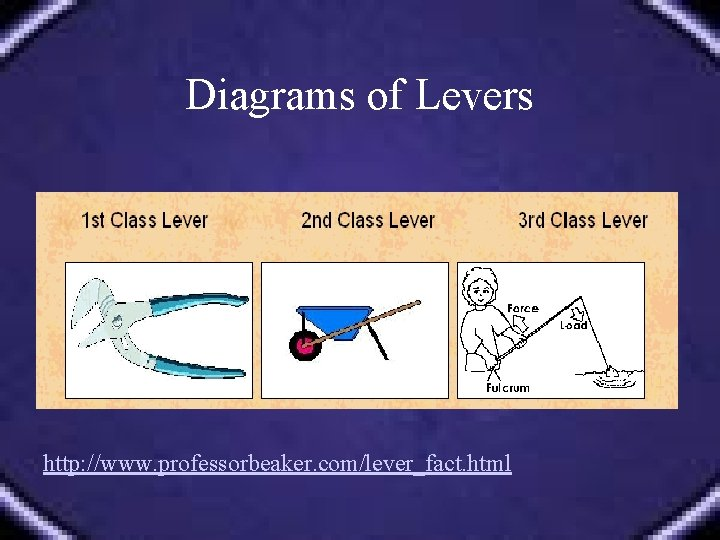Diagrams of Levers http: //www. professorbeaker. com/lever_fact. htmlDescribe PULLEYS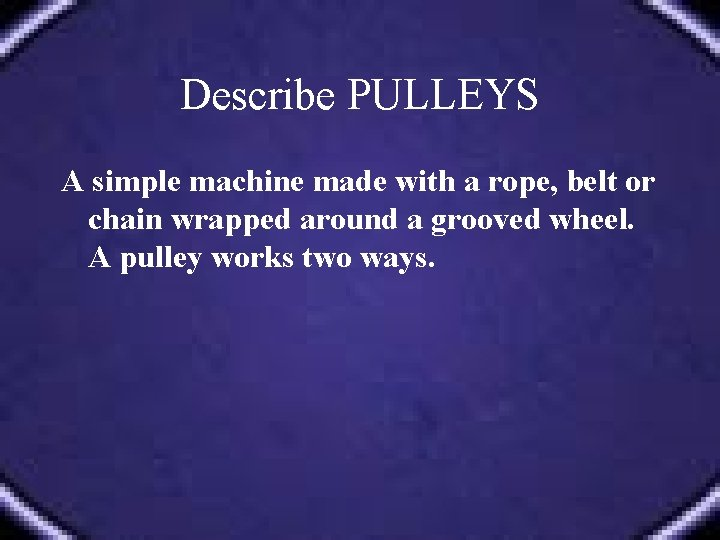Describe PULLEYS A simple machine made with a rope, belt or chain wrapped around a grooved wheel. A pulley works two ways.Diagrams of Pulleys Fixed pulley: Movable Pulley: http: //www. smartown. com/sp 2000/ machines 2000/pulley 2. htm http: //www. smartown. com/sp 2000/ machines 2000/pulley 3. htm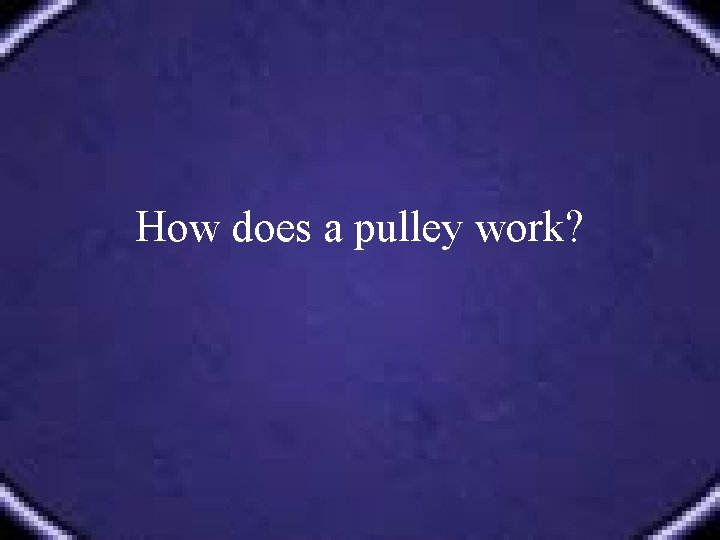How does a pulley work?How does a pulley work? A pulley works two ways. It can change the direction of a force or it can change the amount of force.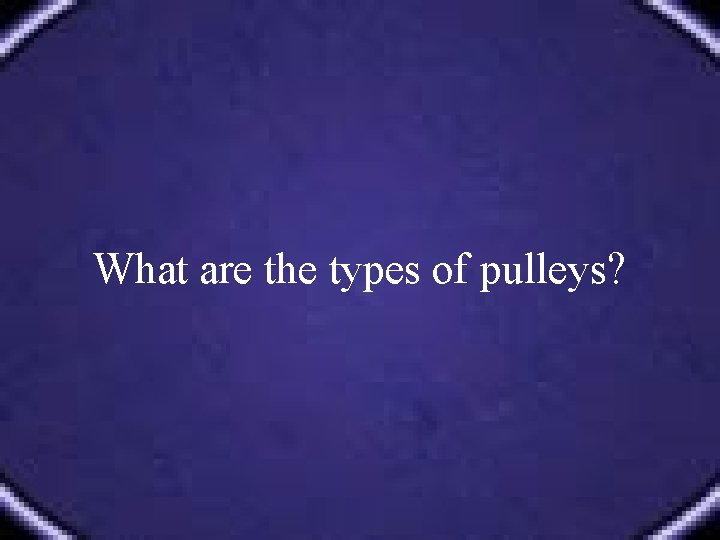What are the types of pulleys?What are the types of pulleys? • Fixed pulley Does not multiply force. Changes the direction of the effort force. Mechanical advantage is equal to one. • Movable pulley Multiplies effort force but cannot change direction of the effort force. Mechanical advantage is the effort distance divided by the resistance distance. • Pulley system A combination of fixed and movable pulleys. Mechanical advantage is equal to the number of supporting ropes.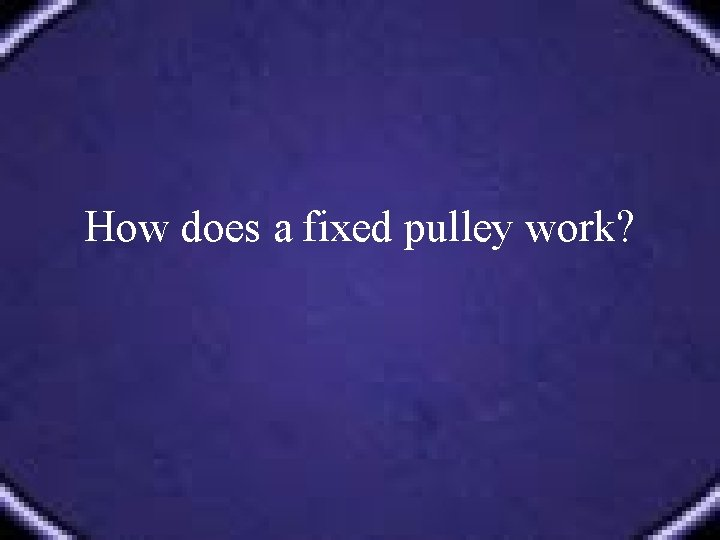How does a fixed pulley work?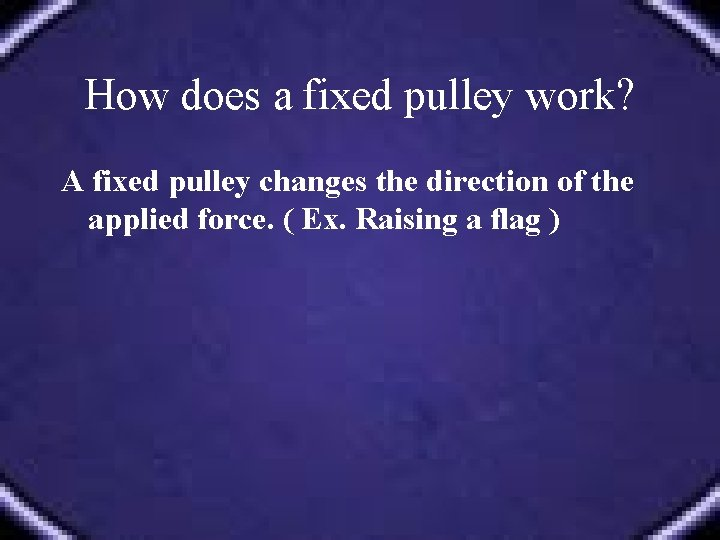How does a fixed pulley work? A fixed pulley changes the direction of the applied force. ( Ex. Raising a flag )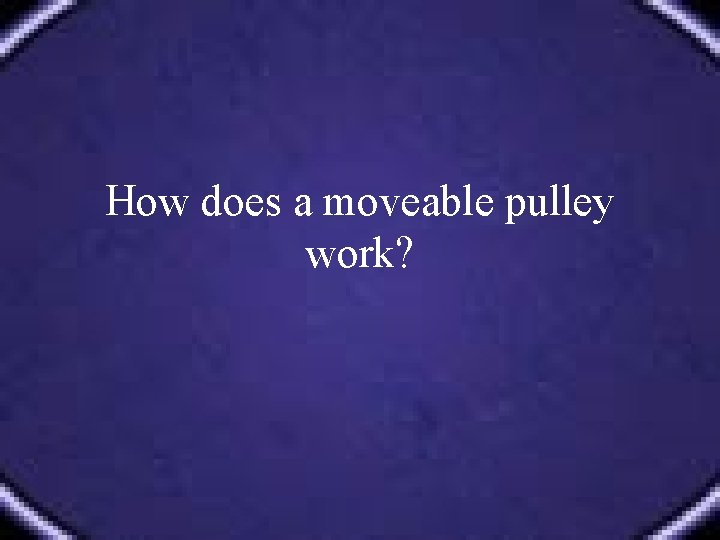How does a moveable pulley work?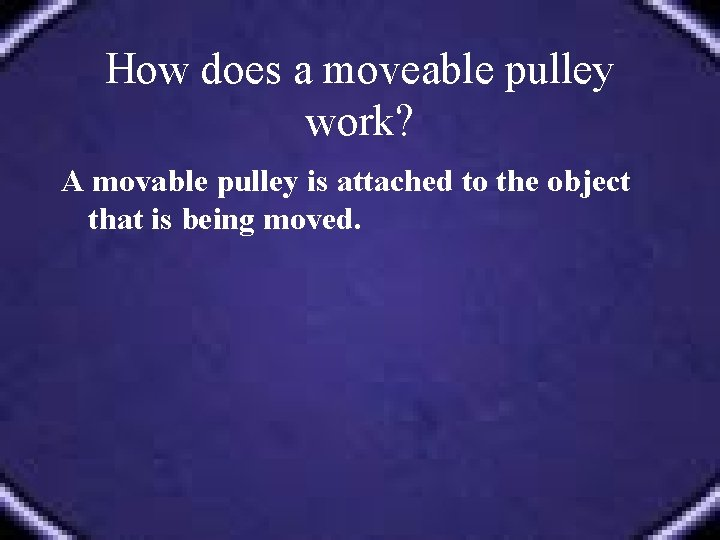How does a moveable pulley work? A movable pulley is attached to the object that is being moved.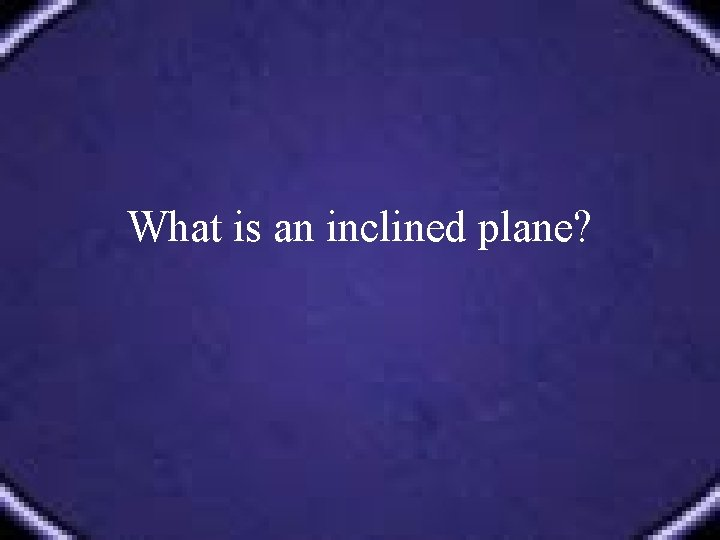What is an inclined plane?What is an inclined plane? A simple machine with no moving parts. It is simply a straight slanted surface. ( Ex. a ramp. )Diagram of Inclined Plane http: //www. smartown. com/sp 2000/machines 2000/inclinedplane. htmWhat is a wheel and axle?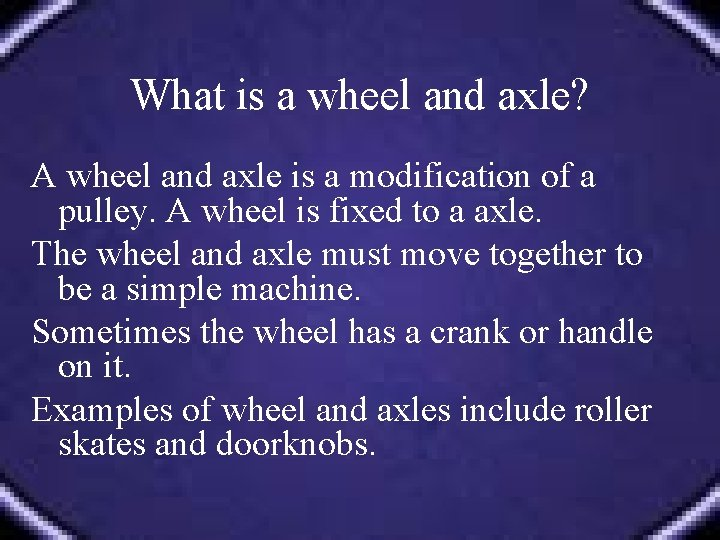What is a wheel and axle? A wheel and axle is a modification of a pulley. A wheel is fixed to a axle. The wheel and axle must move together to be a simple machine. Sometimes the wheel has a crank or handle on it. Examples of wheel and axles include roller skates and doorknobs.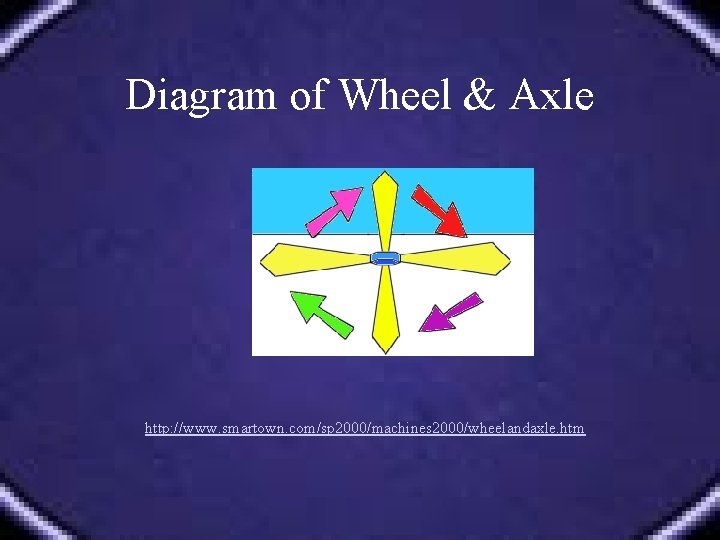Diagram of Wheel & Axle http: //www. smartown. com/sp 2000/machines 2000/wheelandaxle. htm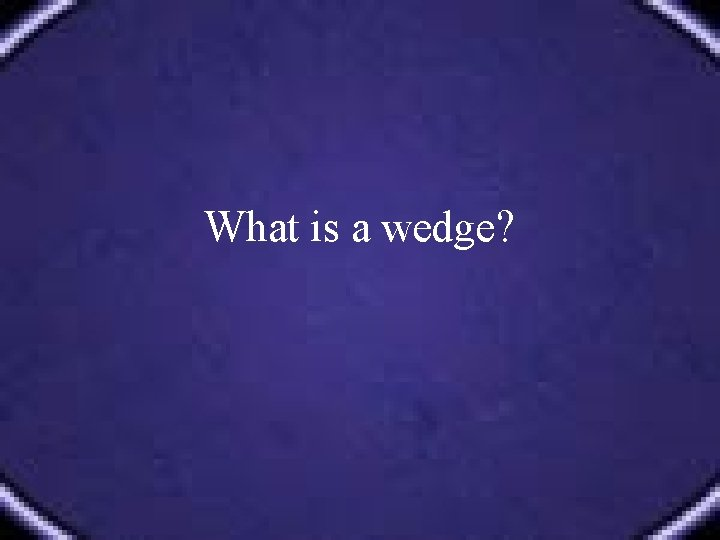What is a wedge?What is a wedge? A modification of an inclined plane that moves. It is made of two inclined planes put together. Instead of the load being moved up an inclined plane, the inclined plane moves the load.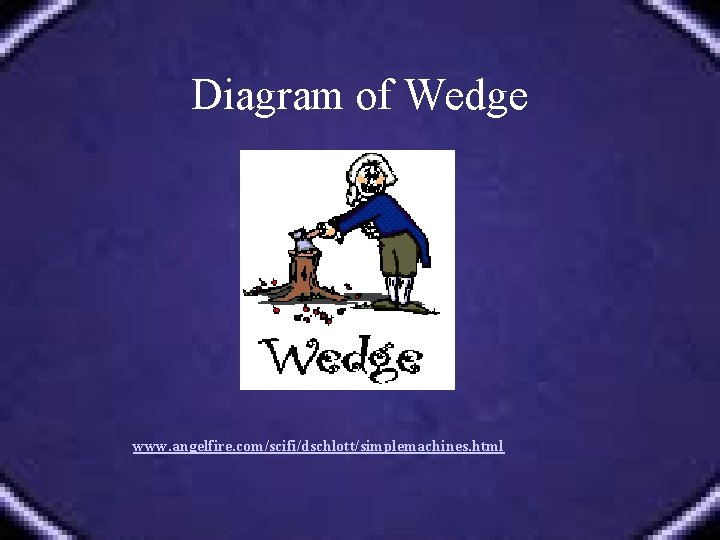Diagram of Wedge www. angelfire. com/scifi/dschlott/simplemachines. html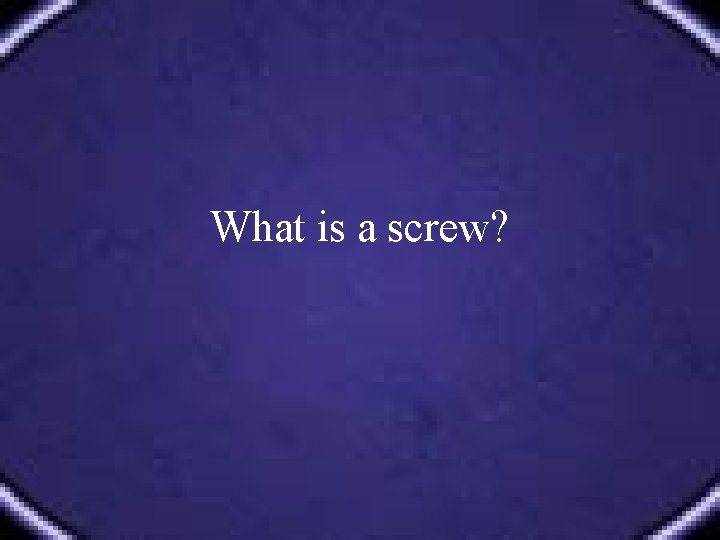What is a screw?What is a screw? A simple machine that is like an inclined plane. It is an inclined plane that wraps around a shaft.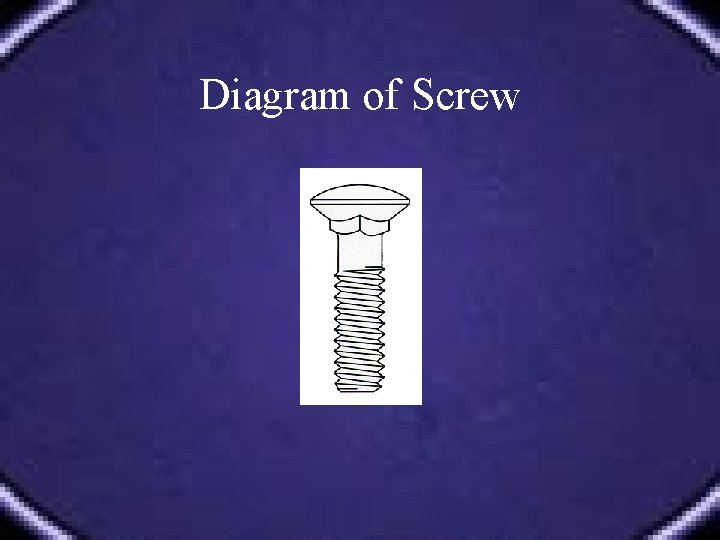Diagram of Screw